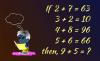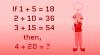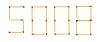BRAIN TEASERSBrain Teasers User Profile

 Mathematical Puzzle: If 2+7=... Mathematical Puzzle: If 2+7=63, 3+2=10, 4+8=96, 5+6=66 then, 9+5=?Calculate 4+20 If 1+5=18, 2+10=36, 3+15=54 then, 4+20=?What is the highest number y... What is the highest number you can make by moving only 2 matchsticks?